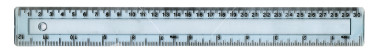WORKSHEET:DECIMALS

Worksheets Descargar PDF
1) Write the numbers:

 a) Five tenths → 0.5 b) Six thousandths c)Twenty hundredths d) Twelve thousandths e) Eighteen ones and two hundredths f) Seven hundredths g) Three ones and seven tenths h) Nine tens and nine tenths i) Forty-five thousandths j) Fifteen millionths

Solution
2) Read and write the following numbers:

 a)0.008 → eight thousandths b) 0.17 c) 6.6 d) 0.05 e) 0.000008 f) 12.3 g) 200.15 h) 0.002 i) 7.000007 j) 3.018

Solution
3) Arrange in ascending order:

a) 3.12, 3.121, 3.11, 3.1212, 3, 3.122, 3.1211b) 0.001, 0.0001, 0.0011, 0.00111, 0.00011, 0.000111, 0.01

Solution
4) Complete with a number:

 a) 2.5 < 2.6 < 2.7 b) 1.7 <         < 1.9 c) 13.04 <         < 13.06 d) 4.12 <         < 4.14 e) 3.113 <        < 3.115 f) 71.14 <        < 71.17 g) 181. 21 <        < 181. 24 h) 3.287 <         < 3.289

Solution
5) Rachel buys 7 chocolates and pays 8.75 . How much does each chocolate cost?Solution
6) Bob had 6.00 . He bought an ice cream for 2.55 and a bag of chips for 0.75 . How much money did he have left?Solution
7) There are 2.54 centimetres in one inch. How many inches are there in 58.42 centimetres?Solution
8) If 5.3 pounds of meat cost \$45.05 what is the price of one pound of meat?Solution
9) Fernando Alonso can travel at 167.35 mph in his McLaren Formula 1 car. How far can he go in 4 hours?Solution
10) Ted was 88.53 cm tall when he was 3 years old. By the time he was 19, Ted had grown a further 89.92 cm. How tall was he when he was 19?Solution